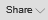# Black and schole model

##### Dhanashre View Profile My Other Post
Costing

Dear All,

Please guide how to calculate the value of N(d1) in following example -

 Number of S.D. from Mean, (z) Area of the left or right (one tail) 0.25 0.4013 0.3 0.3821 0.55 0.2912 0.6 0.2578

Price of stock now = Rs.80
Exercise price = Rs.75
Standard deviation of continuously compounded annual
return = 0.40
Maturity period = 6 months
Annual interest rate = 12%

d1 = 0.5187
How to calculate value of N(d1) ?

Associate

### N(d1)=.74

##### Dhanashre View Profile My Other Post
Costing

How to find out this value

##### john_laphadi View Profile My Other Post
Accounts-Executive

To Dhanshree ,

i would like to give few info. on this question before  further solving :-

1 . This question is already asked in CWA- JUNE 2007 for 8 marks.

2.  ur info in above question is wrong realted to Z Area of  0.6 . The correct proability i.e 0.6 = 0.2743

3.  ur d1 value is also wrong . The correct value of d1 after solving is d1 = 0.58168.

calculation of  N(d1)  = N(.58168) = ?

given table value for (Z = 0.55) = .2912

(Z = 0.60) = .2743

N(0.55) = 1- 0.2912 = .7088 (Refer explanation no. 1)

N(0.60) = 1- 0.2743 = .7257 (Refer explanation no. 1)

Explanation No. 1

as we know that total probability of table is 1 & in "one tail table" if z = +ve then it shows right side area probability of calculated  Z (use right tail table).

now use interpolation technique in figures given below :-

.55       = 0.7088

.58168 = ?

.60        = .07257

from calculation using interpolation technique ,

we got value of N(d1) i.e. N(.58168) = 0.7195.

so , Dhanshree ur query has been solved out .. hope that further part of question will be done by your self ..

Thanks

Total likes : 2 times

##### Dhanashre View Profile My Other Post
Costing(Guest)

Ist Method

Solving by intrapolation method for above problem: As shown by Mr Lapadi

.55 = 0.7088

0.60 = 0.7257

0.5817= ?

By Intrapolation:

[(0.5817-0.5500) / (0.60 - 0.55)] X [0.7257 - 0.7088]

= [0.0317/0.05] X 0.0169

= 0.0107146

Hence N(.5817) = 0.7088 + 0.0107146 = 0.7195. This is the same as Mr  Laphadi has shown

IInd Method : Or you can use the table

d1 = 0.5817. See the table for 0.5 under 8 and that is equal to  0.7190.

Then 0.17 x (0.7224-0.7190) = 0.000578    ( Note: 0.7224 is the value in table after 0.7190)

Then Add 0.7190 + 0.000578 and you get 0.7195.

Regards

John John

##### Paramjeet Singh Gulati View Profile My Other Post
Assistant

Thanks Mr. J LAPHADI.... I searched the same query...now solved .

##### rajat View Profile My Other Post
Student

If two tail area was given then how to calculate nd1

CCI Online Learning

## Choose from our category of courses

What do you want to learn today?

#### CMA

Browse all Subjects

×